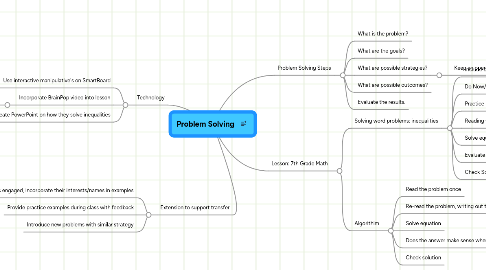# Problem Solving

Get Started. It's FreeProblem Solving## 1. Problem Solving Steps

### 1.3. What are possible strategies?

1.3.1. Keep an open mind for different possibilities!

## 2. Lesson: 7th Grade Math

### 2.1. Solving word problems: inequalities

2.1.1. Include objective/aim of lesson

2.1.2. Do Now/Prior Knowledge: include examples of writing out inequalities

2.1.3. Practice Solving inequalities

2.1.4. Reading word problem, one sentence at a time, write out mathematical statements into an solvable equation

2.1.5. Solve equation

2.1.7. Check Solution

### 2.2. Algorithim

2.2.2. Re-read the problem, writing out the information into a solvable equation

2.2.3. Solve equation

2.2.5. Check solution

## 3. Technology

### 3.2. Incorporate BrainPop video into lesson

3.2.1. http://www.brainpop.com/math/algebra/solvinginequalities/index.weml

### 3.3. Have students work in pairs to create PowerPoint on how they solve inequalities

3.3.1. *Also provides assessment